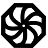# Programming-Idioms# 167
Implementation
Ruby

Be concise.

Be useful.

All contributions dictatorially edited by webmasters to match personal tastes.

Please try to avoid dependencies to third-party libraries and frameworks.

Implementation edit is for fixing errors and enhancing with metadata.

Instead of changing the code of the snippet, consider creating another Ruby implementation.

Other implementations
`import "strings"`
`t := strings.TrimPrefix(s, p)`
`t = s.sub(/\A#{p}/, "")`
`uses StrUtils;`
```if AnsiStartsStr(p, s) then
t := copy(s, length(p)+1, length(s)-length(p))
else
s := t;```
`import std.string;`
`string t = s.chompPrefix(p);`
`let t = s.trim_left_matches(p);`
`t = s[s.startswith(p) and len(p):]`
```import qualified Data.Maybe as Maybe
import qualified Data.List as List```
`t = Maybe.fromMaybe s \$ List.stripPrefix p s`
`#include <string.h>`
```size_t l = strlen(p);
const char * t = strncmp(s, p, l) ? s : s + l;```
```if (s.indexOf(p) === 0) {
t = s.substring(p.length);
}```
`t = s.lstrip(p)`
`\$t = strpos(\$s, \$p) === 0 ? substr(\$s, strlen(\$p)) : \$s;`
```if (0 == index \$s, \$p) {
my \$t = substr \$s, length \$p;
}
```
```  if (index(s,p) == 1) then
t = s(len(p)+1:)
else
t = s
end if```
`var t = s.TrimStart(p);`
`String t = s.replaceFirst("^" + p, "");`